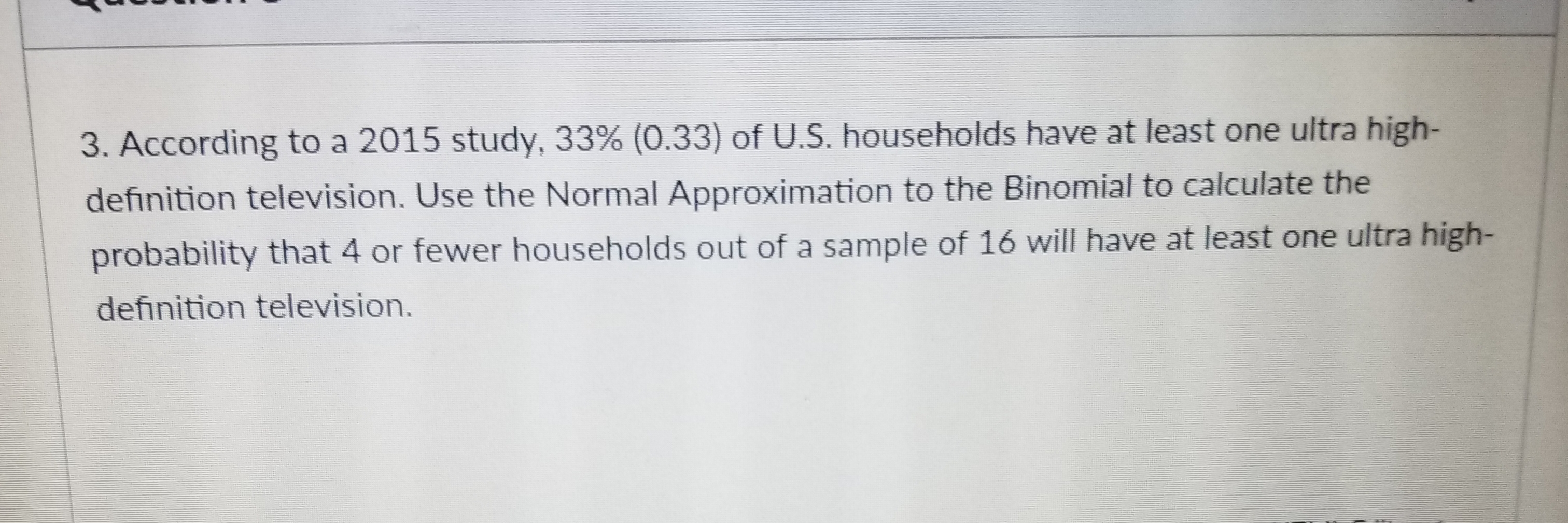# 3. According to a 2015 study, 33% (033) of US, households have at least one ultra high-definition television. Use the Normal Approximation to the Binomial to calculate theprobabilitdefinition television.y that 4 or fewer households out of a sample of 16 will have at least one ultra high-

Question
1 viewshelp_outlineImage Transcriptionclose3. According to a 2015 study, 33% (033) of US, households have at least one ultra high- definition television. Use the Normal Approximation to the Binomial to calculate the probabilit definition television. y that 4 or fewer households out of a sample of 16 will have at least one ultra high- fullscreen
check_circle

Step 1

Introduction to binomial distribution:

Consider a random experiment involving n independent trials, such that the outcome of each trial can be classified as either a “success” or a “failure”. The numerical value “1” is assigned to each success and “0” is assigned to each failure.

Moreover, the probability of getting a success in each trial, p, remains a constant for all the n trials. Denote the probability of failure as q. As success and failure are mutually exclusive, q = 1 – p.

Let the random variable X denote the number of successes obtained from the n trials. Thus, X can take any of the values 0,1,2,…,n.

Then, the probability distribution of X is a Binomial distribution with parameters (n, p) and the probability mass function (pmf) of X, that is, of a Binomial random variable, is given as:

Step 2

Parameters of binomial distribution for the given situation:

The size of the sample taken from the population of U.S households is n = 16. It is known that each and every household will not depend on the other, imp-lying independence among each other. As a result, the 16 households may be considered as 16 independent trials.

Consider the event that house hold having at least one ultra high-definition television as a “success”. It is given that 33% of U.S households have at least one ultra high-definition television. Thus, the probability that house hold having at least one ultra high-definition television, that is, the probability of success in each trial is p = 0.33. Then q = 1 – 0.33 = 0.67.

Consider x as the number of house hold having at least one ultra high-definition television among a sample of 16 U.S households. Then, x has a Binomial distribution with parameters (n = 16, p = 0.33) with pdf as given below:

P(x) = 16Cx*(0.33)x * (0.67)16–x ; x = 0, 1 , 2 , ...,16.

Step 3

Normal approximation to binomial distribution:

The mean and variance for the binomial variate is E(x) = n * p and V(x) = n *p *(1 – p).

Here, p = 0.33, 1 – p = 0.67 and n = 16.

The mean and variance of the variable x (number of house hold having at least one ultra high-definition television) is E(x) = n * p = 16*0.33 = 5.28 and V(x)...

### Want to see the full answer?

See Solution

#### Want to see this answer and more?

Solutions are written by subject experts who are available 24/7. Questions are typically answered within 1 hour.*

See Solution
*Response times may vary by subject and question.
Tagged in

### Other